(Time-to-Event) Survival Analysis

Example Codes:

Survival analysis, also known as time-to-event analysis, refers to the methods of analyzing the expected time until the occurrence of an event. The time-to-event data records the time duration from the start of an observation till an event, the end of the study, lost contact of the participant or withdrawal from the study. Survival analysis involves modelling the time-to-event data. In the context of survival analysis, death and failure are considered as an event, and for each individual the event occurs at most once.

Survival analysis aims to analyze the patterns of event time and solve the relationship between explanatory variables and survival time. There are three common approaches to model the survival function: non-parametric (e.g. Kaplan-Meier method), semi-parametric (e.g. Cox proportional hazards models) and parametric (e.g. Weibull model, log-normal model).

Concepts of common terms

The followings are some common terms in the approaches of survival analysis:

-     Survival function: Probability of surviving past a given time (event-free past t).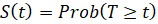-    Probability density function: Unconditional probability that event will occur at the exact time between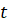and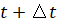: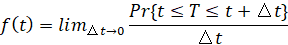-    Hazard function: Instantaneous risk that an event will occur at a given time, given no event until that time: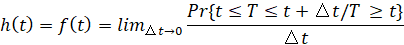Non-parametric analysis

The most common non-parametric technique is the Kaplan-Meir (KM) estimator. The KM estimator breaks the estimation of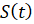into intervals upon observed event times. Within each interval, the probability of surviving is calculated, given that the subjects involved are at risk at the beginning of the time interval.

Under this approach,is the product of survival probabilities at each interval until time.

The estimator of survival probability at each interval is calculated as: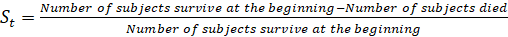This figure shows an example of the survival curve calculated from the KM method.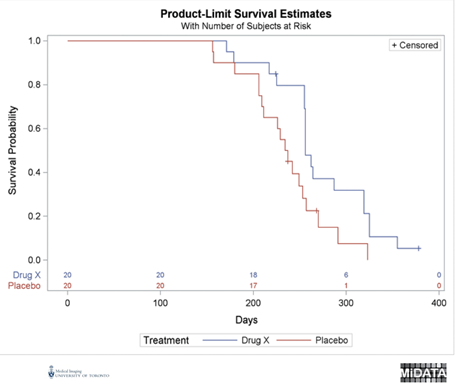Parametric analysis

Compared to non-parametric approaches, parametric forms are more informative and have more statistical power when the models are correctly specified. The hazard function and the effect of the covariates are defined in parametric approaches, where the hazard function is the estimation from an assumed distribution in the underlying population.

Accelerated Failure Time (AFT) models are a class of parametric survival models that can be linearized by taking logs of the survival time model. The AFT model is expressed as: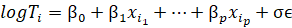where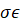is the error term.

The distributions that can specify for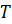and the error term include:

 Distribution of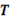Distribution of the error term Weibull Extreme value (2 parameters) Exponential Extreme value (1 parameter) Gamma Log gamma Log-logistic Logistic Log-normal Normal

Example Code in SAS

/* Parametric way */

OPTIONS PS=65 LS=100 NODATE NONUMBER ;

INPUT MINUTES GROUP CENSOR @@ ; DATALINES ;

11 1 0 12 1 0 19 1 0 19 1 0 19 1 0 19 1 0 21 1 0

20 1 0 21 1 0 21 1 0 20 1 0 21 1 0 20 1 0 21 1 0

25 1 0 27 1 0 30 1 0 14 2 0 16 2 0 16 2 0 21 2 0

21 2 0 23 2 0 23 2 0 23 2 0 23 2 0 23 2 0 24 2 0

24 2 0 30 2 0 21 1 1 24 1 1 25 2 1 26 2 1 32 2 1

30 2 1 32 2 1 20 2 1

RUN ;

PROC LIFEREG DATA = HEADACHE ;

CLASS GROUP ;

MODEL MINUTES * CENSOR( 1 ) = GROUP ;

RUN ;

/* Non-parametric way */

PROC FORMAT ; VALUE RX 1 = "DRUG X" 0 ="PLACEBO" ; RUN ;

DATA EXPOSED ; INPUT DAYS STATUS TREATMENT SEX \$ @@ ;

FORMAT TREATMENT RX. ; DATALINES ;

179 1 1 F 378 0 1 M 256 1 1 F 355 1 1 M 262 1 1 M

319 1 1 M 256 1 1 F 256 1 1 M 255 1 1 M 171 1 1 F

224 0 1 F 325 1 1 M 225 1 1 F 325 1 1 M 287 1 1 M

217 1 1 F 319 1 1 M 255 1 1 F 264 1 1 M 256 1 1 F

237 0 0 F 291 1 0 M 156 1 0 F 323 1 0 M 270 1 0 M

253 1 0 M 257 1 0 M 206 1 0 F 242 1 0 M 206 1 0 F

157 1 0 F 237 1 0 M 249 1 0 M 211 1 0 F 180 1 0 F

229 1 0 F 226 1 0 F 234 1 0 F 268 0 0 M 209 1 0 F

RUN ;

ODS GRAPHICS ON ;

TITLE1 “ FIRST OF 3 ANALYSES “ ;

PROC LIFETEST DATA = EXPOSED plots=(survival(atrisk=0 to 1000 by 100 test)

loglogs

logsurv); TIME DAYS * STATUS(0) ; STRATA TREATMENT ; RUN ;

ODS   GRAPHICS   OFF  ;

Example Code in R

install.packages("survival")

install.packages("ranger")

install.packages("ggplot2")

install.packages("dplyr")

install.packages("ggfortify")

library(survival)

library(ranger)

library(ggplot2)

library(dplyr)

library(ggfortify)

# Non-parametric way

# use attached dataset cancer

data("cancer")

# Kaplan Meier Analysis

km_fit <- survfit(Surv(time, status) ~ 1, data=cancer)

summary(km_fit)

autoplot(km_fit)

# Semi-parametric way

vet <- mutate(cancer, AG = ifelse((age < 60), "LT60", "OV60"),

AG = factor(AG))

# Cox proportional hazards model

cox <- coxph(Surv(time, status) ~ 1, data=vet)

summary(cox)

cox_fit <- survfit(cox)

autoplot(cox_fit)

References

1.     Stel V, S, Dekker F, W, Tripepi G, Zoccali C, Jager K, J: Survival Analysis I: The Kaplan-Meier Method. Nephron Clin Pract 2011;119:c83-c88. doi: 10.1159/000324758

2.     Kartsonaki, C. (2016a). Survival analysis. Diagnostic Histopathology, 22(7), 263–270. https://doi.org/10.1016/j.mpdhp.2016.06.005.

3.     Singh, R., & Mukhopadhyay, K. (2011). Survival analysis in clinical trials: Basics and must know areas. Perspectives in clinical research, 2(4), 145–148. https://doi.org/10.4103/2229-3485.86872

4.     Goel, M. K., Khanna, P., & Kishore, J. (2010). Understanding survival analysis: Kaplan-Meier estimate. International journal of Ayurveda research, 1(4), 274–278. https://doi.org/10.4103/0974-7788.76794

5.     Time-To-Event Data Analysis. (n.d.). Columbia Public Health. Retrieved November 3, 2021, from https://www.publichealth.columbia.edu/research/population-health-methods/time-event-data-analysis